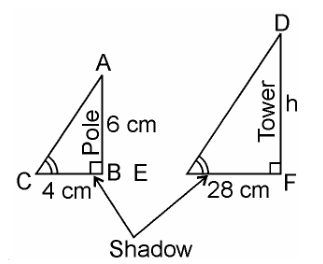# A vertical pole of a length 6 m casts a shadow 4m long on the ground and at the same time a tower casts a shadow 28 m long. Find the height of the tower.

Given,

Length of the vertical pole = 6m

Shadow of the pole = 4 m

Length of shadow of the tower = 28 m

To Prove

Let Height of tower = h m

SolutionFrom ΔABC and ΔDEF,

∠C = ∠E (angular elevation of sum)

∠B = ∠F = 90°

∴ ΔABC ~ ΔDEF (AA similarity criterion)

Hence, AB/DF = BC/EF (If two triangles are similar corresponding sides are proportional)

So we can represent it in equation as

6/h = 4/28

⇒h = (6×28)/4

⇒ h = 6 × 7

⇒ h = 42 m

Hence, the height of the tower is 42 m.